Courses

# Test: Electromagnetic Induction (Competition Level 1)

## 30 Questions MCQ Test Physics Class 12 | Test: Electromagnetic Induction (Competition Level 1)

Description
This mock test of Test: Electromagnetic Induction (Competition Level 1) for Class 12 helps you for every Class 12 entrance exam. This contains 30 Multiple Choice Questions for Class 12 Test: Electromagnetic Induction (Competition Level 1) (mcq) to study with solutions a complete question bank. The solved questions answers in this Test: Electromagnetic Induction (Competition Level 1) quiz give you a good mix of easy questions and tough questions. Class 12 students definitely take this Test: Electromagnetic Induction (Competition Level 1) exercise for a better result in the exam. You can find other Test: Electromagnetic Induction (Competition Level 1) extra questions, long questions & short questions for Class 12 on EduRev as well by searching above.
QUESTION: 1

### A flux of 1m Wb passes through a strip having an area A = 0.02 m2. The plane of the strip is at an angle of 60º to the direction of a uniform field B. The value of B is

Solution: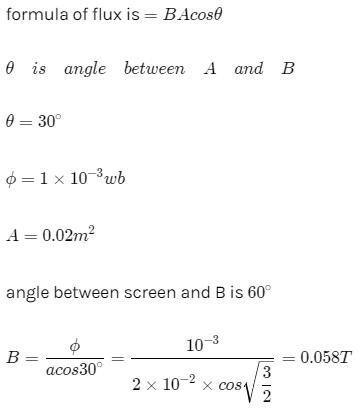QUESTION: 2

### The number of turns in a long solenoid is 500. The area of cross-section of solenoid is 2 × 10-3 m2. If the value of magnetic induction, on passing a current of 2 amp, through it is 5 × 10-3 tesla, the magnitude of magnetic flux connected with it in webers will be

Solution:

Φ=BAN
=5x10-3x2x10-3x500
=5x10-3x1000x10-3
=5x10-3

QUESTION: 3

### A conducting loop of radius R is present in a uniform magnetic field B perpendicular the plane of the ring. If radius R varies as a function of time `t', as R = R0 + t. The e.m.f induced in the loop is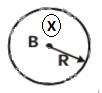Solution:

ε=− dϕ​/dt
=−β(dA/dt)

​⇒∣ε∣=βπd(R0​+t)2
=2πβ(R0​+t)
Since inward flux is increasing the EMF will be induced counter clockwise.

QUESTION: 4

The instantaneous flux associated with a closed circuit of 10Ω resistance is indicated by the following reaction f = 6t2 _ 5t + 1, then the value in amperes of the induced current at t = 0.25 sec will be

Solution:

ϕ=6t2−5t+1
e= dϕ​/dt =12t−5
at t=0.25sec
∣e∣=(12/4−5)=∣3−5∣=2
i=e/R=2/10=0.2A

QUESTION: 5

When a magnet is being moved towards a coil, the induced emf does not depend upon

Solution:

The magnitude of induced emf is independent of the resistance of the coil.
∴e=−dϕ/dt=−N (d/dt) [(BA)/dt]
Thus, e depends upon N, B and A.
But induced current depends upon R.

QUESTION: 6

An electron is moving in a circular orbit of radius R with an angular acceleration a. At the centre of the orbit is kept a conducting loop of radius r, (r < < R). The e.m.f induced in the smaller loop due to the motion of the electron is

Solution:
QUESTION: 7

A field of 5 x 104/p ampere-turns/metre acts at right angles to a coil of 50 turns of area 10-2 m2. The coil is removed from the field in 0.1 second. Then the induced emf in the coil is

Solution: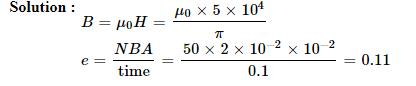QUESTION: 8

square wire loop of 10.0 cm side lies at right angles to a uniform magnetic field of 20T. A 10 V light bulb is in a series with the loop as shown in the fig. The magnetic field is decreasing steadily to zero over a time interval Dt. The bulb will shine with full brightness if Dt is equal to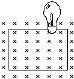Solution:

e=−Δϕ/Δt=ϕi−ϕf/Δt
δt=(ϕi−ϕf)/e=(BA−0)/e=(20×10−2)/10
=20×10−3sec=20ms

QUESTION: 9

The rate of change of magnetic flux denisty through a circular coil of area 10-2 m2 and number of turns 100 is 103 Wb/m2/s. The value of induced e.m.f. will be

Solution: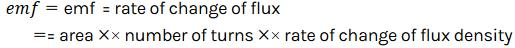QUESTION: 10

A long solenoid contains 1000 turns/cm and an alternating current of peak value 1A is flowing in it. A search coil of area of cross-section 1 × 10-4 m2 and having 50 turns is placed inside the solenoid with its plane perpendicular to the axis of the solenoid. A peak voltage of 2p2 × 10-2V is produced in the search coil. The frequency of current in the solenoid will be

Solution:
QUESTION: 11

In an iron cored coil the iron core is removed so that the coil becomes an air cored coil. The inductance of the coil will

Solution:

In an iron cored coil the iron core is removed so that the coil becomes an air cored coil. The inductance of the coil will decrease.

QUESTION: 12

The magnetic flux through a stationary loop with resistance R varies during interval of time T as f = at (T _ t). The heat generated during this time neglecting the inductance of loop will be

Solution:

E=dx/dt =d(a(Tt-t2))/dt=a(T-2t)
I=E/R=a(T-2t)/R
Heat liberated,
△k=I2Rdt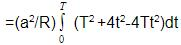=(a2 /R)[T2t+(4t3/3)-2Tt2]T0
=△H=a2T3/3R

QUESTION: 13

The dimensions of permeability of free space can be given by

Solution:

In SI units, permeability is measured in Henries per meter H/m or Hm−1.
Henry has the dimensions of [ML2T−2A−2].
Dimensions for magnetic permeability will be [ML2T−2A−2]/[L]=[MLT−2A−2]

QUESTION: 14

A closed planar wire loop of area A and arbitrary shape is placed in a uniform magnetic field of magnitude B, with its plane perpendicular to magnetic field. The resistance of the wire loop is R. The loop is now turned upside down by 180° so that its plane again becomes perpendicular to the magnetic field. The total charge that must have flowen through the wire ring in the process is

Solution:

Induced emf= d(flux)/dt​=R[dq​/dt]
⇒Δq= Δ(flux)​/R=2BA/R​

QUESTION: 15

A square coil ABCD is placed in x-y plane with its centre at origin. A long straight wire, passing through origin, carries a current in negative z-direction. Current in this wire increases with time.The induced current in the coil is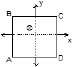Solution:

As magnetic field lines due to current carrying wire is along the surface and hence,
ϕ=B.A=BAcos90O=0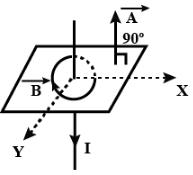QUESTION: 16

Two identical coaxial circular loops carry a current i each circulating in the same direction. If the loops approach each other

Solution:

As the two identical coaxial circular loops carry a current i each circulating in the same direction, their flux add each other. When the two are brought closer, the total flux linkage increases. By Lenz's law a current will be induced in both the loops such that it opposes this change in flux. As the flux increases, the current in each loop will decrease so as to decrease the increasing flux.

QUESTION: 17

In the arrangement shown in given figure current from A to B is increasing in magnitude. Induced current in the loop will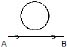Solution:

The direction of the induced current is as shown in the figure, according to Lenz’s law which states that the indeed current flows always in such a direction as to oppose the change which is giving rise to it.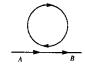QUESTION: 18

The north pole of a magnet is brought near a coil. The induced current in the coil as seen by an observer on the side of magnet will be

Solution:

The direction of the current will be anticlockwise.

According to Lenz's law, the current in the coil will be induced in the direction that'll oppose the external magnetic field .
As the flux due to the external magnet is increasing ( as the N-pole is brought close ) , the coil will have to induce current in the anticlockwise direction to oppose this increase in flux and not in clockwise direction as that'll end up supporting the external flux.

QUESTION: 19

A metal sheet is placed in a variable magnetic field which is increasing from zero to maximum. Induced current flows in the directions as shown in figure. The direction of magnetic field will be -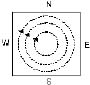Solution:

The direction of these circular magnetic lines is dependent upon the direction of current. The density of the induced magnetic field is directly proportional to the magnitude of the current. Direction of the circular magnetic field lines can be given by Maxwell's right hand grip rule or Right handed cork screw rule.

QUESTION: 20

A small conducting rod of length l, moves with a uniform velocity v in a uniform magnetic field B as shown in fig-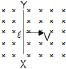Solution:

The rod is moving towards the right in a field directed into the page.
Now, if we apply Fleming's right hand rule, then the direction of induced current will be from end X to end Y.
But, according to Lenz's law the emf induced in the rod will be such that it opposes the motion of the rod.
Hence, the actual emf induced will be from end Y to end X. So, the current will also flow from end Y to end X.
Now, using the convention of current end Y should be positive and end X should be negative.
So, correct answer is option b

QUESTION: 21

The magnetic field in a region is given by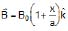. A square loop of edge-length d is placed with its edge along x & y axis. The loop is moved with constant velocity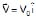. The emf induced in the loop is

Solution: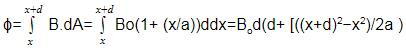By faraday's law
ϵ=− dϕ/dt ​=dϕdx​/dx​dt
=Bo​d (2d​/2a)vo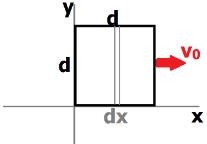QUESTION: 22

Consider the situation shown in fig. The resistanceless wire AB is slid on the fixed rails with a constant velocity. If the wire AB is replaced by a resistanceless semicircular wire, the magnitude of the induced current will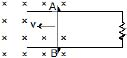Solution:

Rate of change of area with remain same even if wire is replaced by semi-circular wire,
∴ induced current will be same as earlier one as I = (e/R) & e i.e. induced emf is function of area because
e = – N (dɸ/dt) = – N (d/dt) (ABcoswt).

QUESTION: 23

A conducting square loop of side I and resistance R moves in its plane with a uniform velocity v perpendicular to one of its sides. A uniform and constant magnetic field B exists along the perpendicular to the plane of the loop in fig. The current induced in the loop is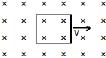Solution:

Voltage is induced due to change in the magnetic flux through a closed loop.
Here
Magnetic Flux = ϕ=BA=const.
Hence
Induced EMF = dtdϕ​=0
Induced current =i=E/R=0

QUESTION: 24

Two identical conductors P and Q are placed on two frictionless fixed conducting rails R and S in a uniform magnetic field directed into the plane. If P is moved in the direction shown in figure with a constant speed, then rod Q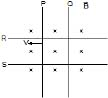Solution:

As the conductor P moves away from Q, the area of the loop enclosed by the conductors and the rails increases. This in turn increases the flux through the loop.
EMF will be induced in such a way that the change in flux will be resisted. The induced current will cause Q to move towards P thereby reducing the area and thus the flux back.

QUESTION: 25

Two infinitely long conducting parallel rails are connected through a capacitor C as shown in the figure. A conductor of length l is moved with constant speed v0. Which of the following graph truly depicts the variation of current through the conductor with time ?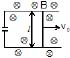Solution:

By Faraday's Law of induction,
ε=− dϕ​/dt
=−Bl (dx/dt) ​=−Blv0​
This emf should induce the movement of charges creating a current. But due to the attached capacitor, all charges are conserved.
Thus I= dq/dt ​=0
The correct option is C.

QUESTION: 26

A thin wire of length 2m is perpendicular to the xy plane. It is moved with velocity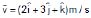through a region of magnetic induction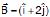Wb/m2. Then potential difference induced between the ends of the wire

Solution: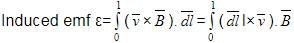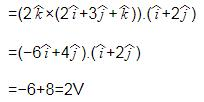QUESTION: 27

A long metal bar of 30 cm length is aligned along a north south line and moves eastward at a speed of 10 ms-1. A uniform magnetic field of 4.0 T points vertically downwards. If the south end of the bar has a potential of 0V, the induced potential at the north end of the bar is

Solution:

Induced emf =Blv=12V. It is induced in the northward direction by right hand rule (emf=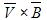)
therefore if the south end of the pole has potential of 0V, the north end will have a potential of 12V.

QUESTION: 28

A conducting rod moves with constant velocity v perpendicular to the long, straight wire carrying a current I as shown compute that the emf generated between the ends of the rod.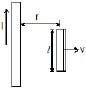Solution:

The magnetic field near the conducting rod due to long current carrying wire is
B= μ0​I/2πr ​ (using Ampere's law, ∫B.dl=μ0​I)
Now, induced emf due to moving rod is e=Blv= (μ0​I/2πr) ​×lv= μ0​vIl/2πr

QUESTION: 29

A square loop of side a and resistance R is moved in the region of uniform magnetic field B (loop remaining completely insidefield), with a velocity v through a distance x. The work done is

Solution: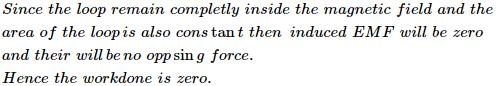QUESTION: 30

A rod closing the circuit shown in figure moves along a U shaped wire at a constant speed v under the action of the force F. The circuit is in a uniform magnetic field perpendicular to the plane. Calculate F if the rate of heat generation in the circuit is Q.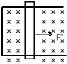Solution: